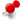# Entity EquationClassicListThreaded2 messagesReply | Threaded
Open this post in threaded view
|

## Entity Equation

 For the quadratic equations, I was thinking to add a equation method to the cadentity class and for that, I'll be first needing to port some maths stuff. Your views over this ?
Reply | Threaded
Open this post in threaded view
|

## Re: Entity Equation

 the lc_quadratic contains an equation in a form of quadratic, namely, {x, y}.m_mQuad.{{x},{y}} + m_vLinear.{{x},{y}}+m_dConst = 0 Can you explain your ideas about equations in details?